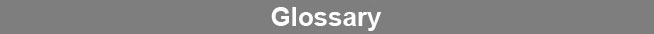Select Page### Chi Square Distribution

Chi square (goodness of fit) distributions are a family of probability distributions of the chi square statistic, one for each degree of freedom. For small degrees of freedom, the distribution is skewed to the left. As the number of degrees of freedom increases, the distribution rapidly becomes symmetrical. For large degrees of freedom, the chi square distribution closely approximates a Gaussian curve.www.brendan.com